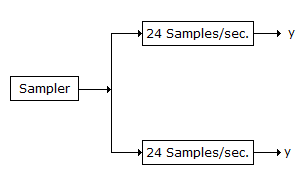# Electronics and Communication Engineering - Exam Questions Papers - Discussion

Discussion Forum : Exam Questions Papers - Exam Paper 10 (Q.No. 36)
36.
In analog signal given below is sampled at such a rate that the samples of signal can be given to a collection of buffers having capacity of either 24 samples/sec. or 25 samples/sec. The sampling rate is minimum such that it fully utilizes the collection of both types of buffers m(t) = 2 sin 480pt + 3 sin 720 pt
The folding (maximum) frequency is
600 Hz
300 Hz
1200 Hz
2400 Hz
Explanation:LCM of 24 and 25 is 600 which will give maximum utilization of the buffers

Sampling frequency is 600

Folding frequency = 600/2 = 300.

Discussion:
Be the first person to comment on this question !